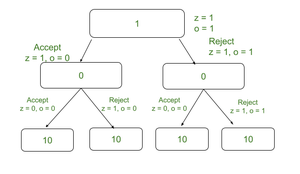Related Articles
Length of longest subset consisting of A 0s and B 1s from an array of strings
• Last Updated : 08 Apr, 2021

Given an array arr[] consisting of N binary strings, and two integers A and B, the task is to find the length of the longest subset consisting of at most A 0s and B 1s.

Examples:

Input: arr[] = {“1”, “0”, “0001”, “10”, “111001”}, A = 5, B = 3
Output: 4
Explanation:
One possible way is to select the subset {arr, arr, arr, arr}.
Total number of 0s and 1s in all these strings are 5 and 3 respectively.
Also, 4 is the length of the longest subset possible.

Input: arr[] = {“0”, “1”, “10”}, A = 1, B = 1
Output: 2
Explanation:
One possible way is to select the subset {arr, arr}.
Total number of 0s and 1s in all these strings is 1 and 1 respectively.
Also, 2 is the length of the longest subset possible.

Naive Approach: The simplest approach to solve the above problem is to use recursion. At every recursive call, the idea is to either include or exclude the current string. Once, all possibilities are considered, print the maximum length of subset obtained.

Below is the implementation of the above approach:

## C++14

 `// C++ program for the above approach``#include ``using` `namespace` `std;`` ` `// Function to count 0's in a string``int` `count0(string s)``{``    ``// Stores count of 0s``    ``int` `count = 0;`` ` `    ``// Iterate over characters of string``    ``for` `(``int` `i = 0; i < s.size(); i++) {`` ` `        ``// If current character is '0'``        ``if` `(s[i] == ``'0'``) {``            ``count++;``        ``}``    ``}``    ``return` `count;``}`` ` `// Recursive function to find the length of``// longest subset from an array of strings``// with at most A 0's and B 1's``int` `solve(vector vec,``          ``int` `A, ``int` `B, ``int` `idx)``{``    ``// If idx is equal to N``    ``// or A + B is equal to 0``    ``if` `(idx == vec.size() || A + B == 0) {``        ``return` `0;``    ``}`` ` `    ``// Stores the count of 0's in arr[idx]``    ``int` `zero = count0(vec[idx]);`` ` `    ``// Stores the count of 1's in arr[idx]``    ``int` `one = vec[idx].size() - zero;`` ` `    ``// Stores the length of the``    ``// subset if arr[i] is included``    ``int` `inc = 0;`` ` `    ``// If zero is less than or equal to A``    ``// and one is less than or equal to B``    ``if` `(zero <= A && one <= B) {``        ``inc = 1 + solve(vec, A - zero,``                        ``B - one, idx + 1);``    ``}`` ` `    ``// Stores the length of the subset``    ``// if arr[i] is excluded``    ``int` `exc = solve(vec, A, B, idx + 1);`` ` `    ``// Returns max of inc and exc``    ``return` `max(inc, exc);``}`` ` `// Function to find the length of the``// longest subset from an array of``// strings with at most A 0's and B 1's``int` `MaxSubsetlength(vector arr,``                    ``int` `A, ``int` `B)``{``    ``// Return``    ``return` `solve(arr, A, B, 0);``}`` ` `// Driver Code``int` `main()``{``    ``vector arr = { ``"1"``, ``"0"``, ``"10"` `};``    ``int` `A = 1, B = 1;`` ` `    ``cout << MaxSubsetlength(arr, A, B);``    ``return` `0;``}`
Output:
```2
```

Time Complexity: O(2N
Auxiliary Space: O(1)

Efficient Approach: The above approach can be optimized using Memoization, based on the following observations:Recursion Tree

• It can be observed that there exists a overlapping subproblem and optimal substructure property.
Therefore, the idea is to use dynamic programming to optimize the above approach.
• The idea is to use the 3D-state dynamic programming.
• Suppose Dp(i, A, B) represents the maximum length of the subset with at most A 0s and B 1s.
• Then the transition of states can be defined by either selecting a string at ith index or not,
• Dp(i, A, B) = Max(1+Dp(i+1, A- Z, B-O), Dp(i+1, A, B)),
where Z is the count of Os and O is the count of 0s.

Below is the implementation of the above approach:

## C++

 `// C++ program for the above approach``#include ``using` `namespace` `std;`` ` `// Function to count number``// of 0s present in the string``int` `count0(string s)``{``    ``// Stores the count of 0s``    ``int` `count = 0;`` ` `    ``// Iterate over characters of string``    ``for` `(``int` `i = 0; i < s.size(); i++) {`` ` `        ``// If current character is '0'``        ``if` `(s[i] == ``'0'``) {``            ``count++;``        ``}``    ``}``    ``return` `count;``}``// Recursive Function to find the length of``// longest subset from given array of strings``// with at most A 0s and B 1s``int` `solve(vector vec, ``int` `A,``          ``int` `B, ``int` `idx,``          ``vector > >& dp)``{``    ``// If idx is equal to N or``    ``// A + B is equal to 0``    ``if` `(idx == vec.size() || A + B == 0) {``        ``return` `0;``    ``}``    ``// If the state is already calculated``    ``if` `(dp[A][B][idx] > 0) {``        ``return` `dp[A][B][idx];``    ``}`` ` `    ``// Stores the count of 0's``    ``int` `zero = count0(vec[idx]);`` ` `    ``// Stores the count of 1's``    ``int` `one = vec[idx].size() - zero;`` ` `    ``// Stores the length of longest``    ``// by including arr[idx]``    ``int` `inc = 0;`` ` `    ``// If zero is less than A``    ``// and one is less than B``    ``if` `(zero <= A && one <= B) {``        ``inc = 1``              ``+ solve(vec, A - zero,``                      ``B - one, idx + 1, dp);``    ``}`` ` `    ``// Stores the length of longest subset``    ``// by excluding arr[idx]``    ``int` `exc = solve(vec, A, B, idx + 1, dp);`` ` `    ``// Assign``    ``dp[A][B][idx] = max(inc, exc);`` ` `    ``// Return``    ``return` `dp[A][B][idx];``}`` ` `// Function to find the length of the``// longest subset of an array of strings``// with at most A 0s and B 1s``int` `MaxSubsetlength(vector arr,``                    ``int` `A, ``int` `B)``{``    ``// Stores all Dp-states``    ``vector > > dp(``        ``A + 1,``        ``vector >(B + 1,``                             ``vector<``int``>(arr.size() + 1,``                                         ``0)));``    ``// Return``    ``return` `solve(arr, A, B, 0, dp);``}`` ` `// Driver Code``int` `main()``{``    ``vector arr = { ``"1"``, ``"0"``, ``"10"` `};``    ``int` `A = 1, B = 1;`` ` `    ``cout << MaxSubsetlength(arr, A, B);``    ``return` `0;``}`
Output:
```2
```

Time Complexity: O(N*A*B)
Auxiliary Space: O(N*A*B)

Attention reader! Don’t stop learning now. Get hold of all the important DSA concepts with the DSA Self Paced Course at a student-friendly price and become industry ready.

My Personal Notes arrow_drop_up